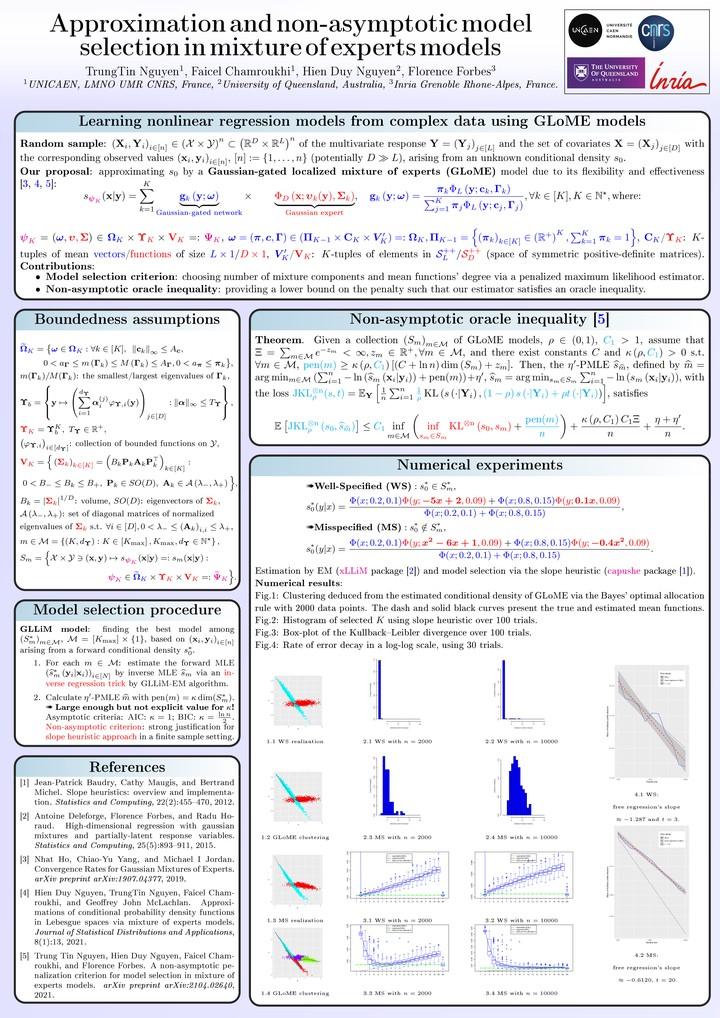# Approximation and non-asymptotic model selection in mixture of experts modelsImage credit: TrungTin Nguyen

### Abstract

Mixtures of experts (MoE) models are a ubiquitous tool for the analysis of heterogeneous data across many fields including statistics, bioinformatics, pattern recognition, economics, and medicine, among many others. They provide conditional constructions for regression in which the mixture weights, along with the component densities, are explained by the predictors, allowing for flexibility in the modeling of data arising from complex data generating processes. In this work, we consider the Gaussian-gated localized MoE (GLoME) regression model for modeling heterogeneous data. This model poses challenging questions with respect to the statistical estimation and model selection problems both from the computational and theoretical points of view. We establish non-asymptotic risk bounds that take the form of weak oracle inequalities, provided that lower bounds on the penalties hold true, in high-dimensional regression scenarios for a variety of MoE regression models, including Gaussian-gated and softmax-gated Gaussian MoE, based on an inverse regression strategy or a Lasso penalization, respectively. In particular, our oracle inequalities show that the performance in Jensen–Kullback–Leibler type loss of our penalized maximum likelihood estimators are roughly comparable to that of oracle models if we take large enough the constants in front of the penalties, whose forms are only known up to multiplicative constants and proportional to the dimensions of models. Such theoretical justifications of the penalty shapes motivate us to make use of the slope heuristic criterion to select several hyperparameters, including the number of mixture components, the amount of sparsity (the coefficients and ranks sparsity levels), the degree of polynomial mean functions, and the potential hidden block-diagonal structures of the covariance matrices of the multivariate predictor or response variable. To support our theoretical results and the statistical study of non-asymptotic model selection in a variety of MoE models, we perform numerical studies by considering simulated and real data, which highlight the performance of our finite-sample oracle inequality results.

Date
Sep 30, 2021 9:00 AM — Sep 29, 2021 5:00 PM
Location
INSA Rouen Normandie, Rouen, France##### TrungTin Nguyen
###### Postdoctoral Fellow

A central theme of my research is data science at the intersection of statistical learning, machine learning and optimization.